Count Data

Example Codes:

Definition

Count data is a statistical data type that the observed values are non-negative integers and are in the form of counts. The count variable is a random variable where Poisson, binomial and negative binomial distributions are commonly used to describe its distribution. Poisson regression can be used to model count variables.

Poisson Regression

The Poisson distribution describes the probability that a random event will occur in a time or space interval when the probability of the event occurring is very small, but the number of trials is very large.

Model

Poisson regression models are generalized linear models with the Poisson distribution function. The log link function is commonly used in the models.

The Poisson probability distribution: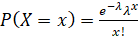The Poisson response variable may be modeled as: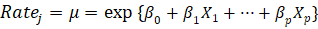Sometimes, the count responses will pertain to unequal units of time or space. In such cases, we let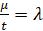. Then we have: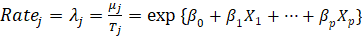Using the Log Link, we obtain: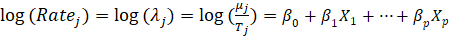Overdispersion

A characteristic of the Poisson distribution is that its mean is equal to its variance. If we see that the observed variance is greater than the mean - this is known as overdispersion. It tells us that the model is not appropriate.

A common reason for overdispersion is the exclusion of relevant explanatory variables.

Example Code in SAS

data insure;

input n c car\$ age;

ln = log(n);

datalines;

500   42  small  1

1200  37  medium 1

100    1  large  1

400  101  small  2

500   73  medium 2

300   14  large  2

;

proc genmod data=insure;

class car age;

model c = car age / dist   = poisson

offset = ln;

run;

Example Code in R

# input data

n <- c(500, 1200, 100, 400, 500, 300)

c <- c(42, 37, 1, 101, 73, 14)

car <- c("small", "medium", "large", "small", "medium", "large")

age <- c(1, 1, 1, 2, 2, 2)

# identify car and age as categorical variables

car <- factor(car)

age <- factor(age)

# create the dataframe

df <- data.frame(n, c, car, age)

# Poisson regression

glm(formula = c ~ car + age + n, data = df, family = poisson(link="log"))

References

1.     Cameron, A. C.; Trivedi, P. K. (2013). Regression Analysis of Count Data Book (Second ed.). Cambridge University Press. ISBN 978-1-107-66727-3.

2.     Poisson Regression. (2019, December 13). SAS® Help Center. https://documentation.sas.com/doc/en/pgmsascdc/9.4_3.3/statug/statug_ttest_syntax01.htm